Courses

# Systems of Linear Equations, Matrix Algebra & Transform Theory - MCQ Test - 2

## 30 Questions MCQ Test Topicwise Question Bank for Electronics Engineering | Systems of Linear Equations, Matrix Algebra & Transform Theory - MCQ Test - 2

Description
This mock test of Systems of Linear Equations, Matrix Algebra & Transform Theory - MCQ Test - 2 for GATE helps you for every GATE entrance exam. This contains 30 Multiple Choice Questions for GATE Systems of Linear Equations, Matrix Algebra & Transform Theory - MCQ Test - 2 (mcq) to study with solutions a complete question bank. The solved questions answers in this Systems of Linear Equations, Matrix Algebra & Transform Theory - MCQ Test - 2 quiz give you a good mix of easy questions and tough questions. GATE students definitely take this Systems of Linear Equations, Matrix Algebra & Transform Theory - MCQ Test - 2 exercise for a better result in the exam. You can find other Systems of Linear Equations, Matrix Algebra & Transform Theory - MCQ Test - 2 extra questions, long questions & short questions for GATE on EduRev as well by searching above.
QUESTION: 1

### Consider the system of equations given below:  x + y =  2 2x + 2y = 5 This system has

Solution:

(b) This can be written as AX = B Where A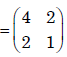Angemented matrix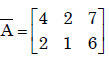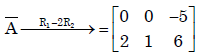rank(A) ≠ rank(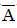). The system is inconsistant .So system has no solution.

QUESTION: 2

### For what value of a, if any, will the following system of equations in x, y and z have a solution?   2x + 3y = 4 x+y+z = 4 x + 2y - z = a

Solution:

(b)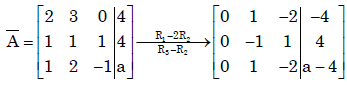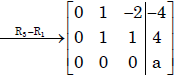If a = 0 then rank (A) = rank() = 2. Therefore the system is consistant
∴ The system has soln .

QUESTION: 3

### Solution for the system defined by the set of equations 4y + 3z = 8; 2x – z = 2 and 3x + 2y =5 is

Solution:

Ans.(d)
Consider the matrix A =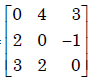,Now det (A) = 0
So byCramer's Rule the system has no solution

QUESTION: 4

For what values of α and β the following simultaneous equations have an infinite numberof solutions?
x + y + z = 5; x + 3y + 3z = 9; x + 2y + αz = β

Solution:

(d)=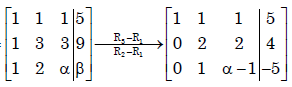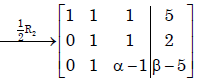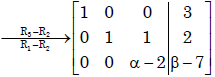For infinite solution of the system
α − 2 = 0 and β − 7 = 0
⇒ α = 2 and β = 7.

QUESTION: 5

Let A be a 3 × 3 matrix with rank 2. Then AX = 0 has

Solution:

(b)
We know , rank (A) + Solution space X(A) = no. of unknowns.
⇒2 + X(A) = 3 . [Solution space X(A)= No. of linearly independent vectors]
⇒ X(A) =1.

QUESTION: 6

A is a 3 x 4 real matrix and A x = b is an inconsistent system of equations. The highest possible rank of A is

Solution:

(b). Highest possible rank of A= 2 ,as Ax = b is an inconsistent system.

QUESTION: 7

Consider the matrices X (4 × 3), Y (4 × 3) and P (2 × 3). The order or P (XTY)–1PT] T will be

Solution:

(a)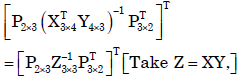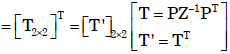QUESTION: 8

Given matrix [A] =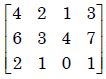the rank of the matrix is

Solution:

(c)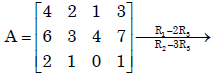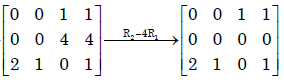∴Rank(A) = 2

QUESTION: 9

The Laplace transform of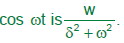Solution:

Ans. (b)False
Laplace transform of

QUESTION: 10

There are two containers, with one containing 4 Red and 3 Green balls and the other containing Blue and 4 Green balls. One bal is drawn at random form each container.The probability that one of the ball is Red and the other is Blue will be

Solution:
QUESTION: 11

If L defines the Laplace Transform of a function, L [sin (at)] will be equal to

Solution:

Ans. (b)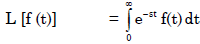⇒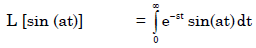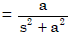QUESTION: 12

The Inverse Laplace transform of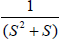is

Solution:

Ans. (c)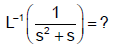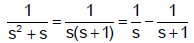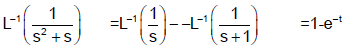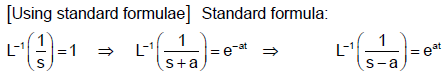QUESTION: 13

Laplace transform for the function f(x) = cosh (ax) is

Solution:

Ans. (b)
It is a standard result that
L (cosh at) =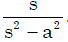QUESTION: 14

If F(s) is the Laplace transform of function f (t), then Laplace transform of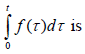Solution:

Ans. (a)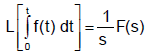QUESTION: 15

Laplace transform of the function sin ωt

Solution:

Ans. (b)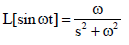QUESTION: 16

Laplace transform of (a + bt)2 where ‘a’ and ‘b’ are constants is given by:

Solution:

Ans.(c)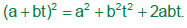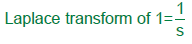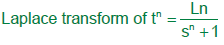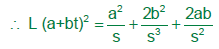QUESTION: 17

A delayed unit step function is defined as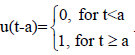Its Laplace transform is

Solution:

Ans. (d)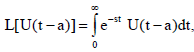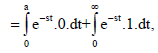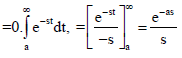QUESTION: 18

The Laplace transform of the function sin2 2t is

Solution:

Ans.(a)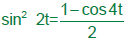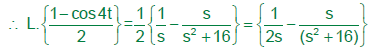QUESTION: 19

Find the rank of the matrix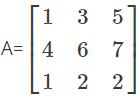Solution:

To find out the rank of the matrix first find the |A|
If the value of the |A| = 0 then the matrix is said to be reduced
But, as the determinant of A has some finite value, then the rank of the matrix is 3.

QUESTION: 20

The running integrator, given by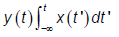Solution:

Ans. (b)

QUESTION: 21

The state transition matrix for the system  X- = AX with initial state X(0) is

Solution:

Ans. (c)

QUESTION: 22

The Fourier transform of x(t) = e–at u(–t), where u(t) is the unit step function

Solution:

Ans. (d)

QUESTION: 23

The fundamental period of the discrete-time signal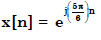is

Solution:

Ans. (b)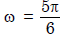or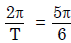or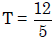QUESTION: 24

u(t) represents the unit step function. The Laplace transform of u(t – ζ) is

Solution:

Ans. (c)
f(t) = u(t – ζ)
L{f(t)} = L{u(t – ζ)}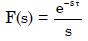QUESTION: 25

The fundamental period of x(t) = 2 sin πt + 3 sin 3πt, with t expressed in seconds, is

Solution:

Ans. (d)
H.C.F. of 2π and 3π is 6π.
Then, fundamental frequency = 6π
∴ Period, T =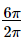= 3 sec

QUESTION: 26

If the Fourier transform of x[n] is X(e), then the Fourier transform of (–1)n x[n] is

Solution:

Ans. (c)

QUESTION: 27

Given f(t) and g(t) as shown below: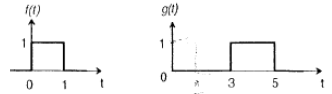g (t) can be expressed as

Solution:
QUESTION: 28

Given f(t) and g(t) as shown below:The Laplace transform of g(t) is

Solution:

Ans. (c)

QUESTION: 29

The Laplace transform of g(t) is

Solution:

Ans. (c)

QUESTION: 30

Let Y(s) be the Laplace transformation of the function y (t), then final value of the function is

Solution:

Ans. (c)